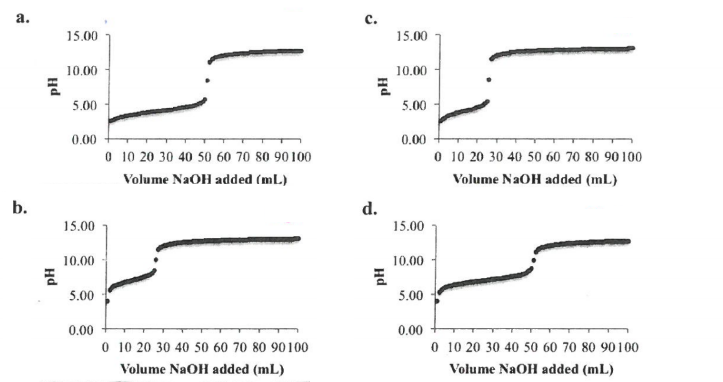# Problem: Suppose you titrated 50mL of a 1.0 M monoprotic acid solution with a 2.0 M NaOH solution. If the Ka of your acid is 1.0 x 10-6, which of the following curves best represents the titration?

88% (14 ratings)
###### Problem Details

Suppose you titrated 50mL of a 1.0 M monoprotic acid solution with a 2.0 M NaOH solution. If the Ka of your acid is 1.0 x 10-6, which of the following curves best represents the titration?What scientific concept do you need to know in order to solve this problem?

Our tutors have indicated that to solve this problem you will need to apply the Acid and Base Titration Curves concept. You can view video lessons to learn Acid and Base Titration Curves. Or if you need more Acid and Base Titration Curves practice, you can also practice Acid and Base Titration Curves practice problems.

What is the difficulty of this problem?

Our tutors rated the difficulty ofSuppose you titrated 50mL of a 1.0 M monoprotic acid solutio...as high difficulty.

How long does this problem take to solve?

Our expert Chemistry tutor, Dasha took 6 minutes and 12 seconds to solve this problem. You can follow their steps in the video explanation above.

What professor is this problem relevant for?

Based on our data, we think this problem is relevant for Professor Sandler's class at NAU.